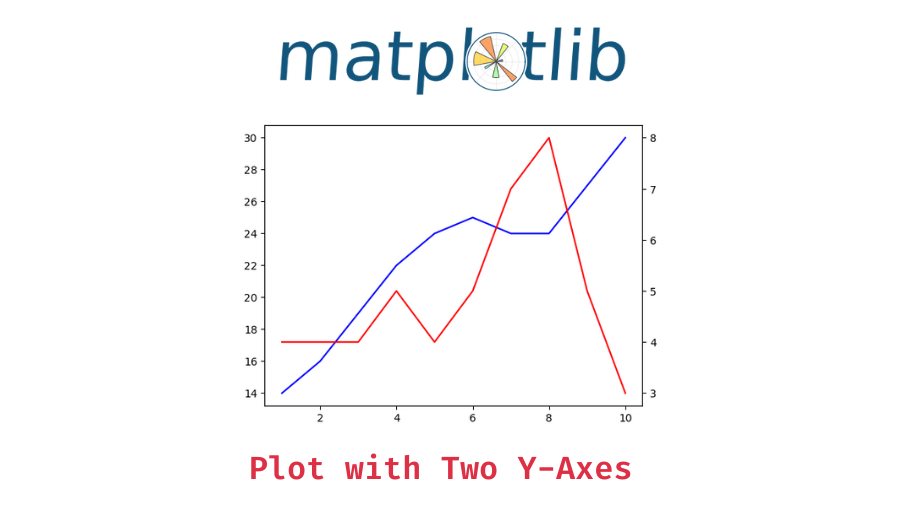# Matplotlib – Create a Plot with two Y Axes and shared X Axis

In this tutorial, we’ll try to understand how to create a matplotlib plot with two Y axes and a shared X axis and why it is used.

You might occasionally want to create a single plot that has two variables with different scales as part of a brief exploratory data study. Making a single plot with two different y-axes — one on the left for one variable and the other on the right for the other y-variable is an alternative that is commonly used.

The plot would not really make sense if we were to present the two variables on the same graph without using two different y-axes. If the variables have very different scales, we’ll want to make sure that we plot them in different twin Axes objects. These objects can share one axis (for example, the time, or x-axis) while not sharing the other (the y-axis).

## How to create a plot with two different Y axes in matplotlib?

You can use the matplotlib `Axes` object’s `twinx()` method to create new Axes with an invisible x-axis and an independent y-axis positioned opposite to the original one (i.e. at right).

Basic Syntax:

`Axes.twinx()`

Returns: The newly created Axes instance

Now, let us understand the above method with some worked-out examples.

### Example 1 – Plot two straight lines with different Y axes

```import matplotlib.pyplot as plt
import numpy as np

#defining X and Y1,Y2 values
x = np.linspace(0,10)
y1 = 2*x+4

y2 = 5-2*x

#setting the figure and axes
fig,ax = plt.subplots()

#plotting the first plot
ax.plot(x,y1,color='b')

#using twinx to generate another y axes and plotting
ax2 = ax.twinx()
ax2.plot(x,y2,color='r')```

Output:

📚 Data Science Programs By Skill Level

Introductory

Intermediate ⭐⭐⭐

🔎 Find Data Science Programs 👨‍💻 111,889 already enrolled

Disclaimer: Data Science Parichay is reader supported. When you purchase a course through a link on this site, we may earn a small commission at no additional cost to you. Earned commissions help support this website and its team of writers.

In the above example, we –

1. Import the required modules.
2. Create the x values using the `np.linspace` method (Refer this).
3. Generate the respective y values for 2 different line equations i.e., `y1=2x+4` & `y2=5-2x`.
4. Create a subplot and plot the y1 values on it.
5. Use the `twinx()` method to create an Axes object with a shared X axis and plot the y2 values to it.

### Example 2 – Plot two trend lines with different Y axes

```import matplotlib.pyplot as plt
import numpy as np

#defining X and Y1,Y2 values
x = [1, 2, 3, 4, 5, 6, 7, 8, 9, 10]
y1 = [14, 16, 19, 22, 24, 25, 24, 24, 27, 30]

y2 = [4, 4, 4, 5, 4, 5, 7, 8, 5, 3]

#setting the figure and axes
fig,ax = plt.subplots()

#plotting the first plot
ax.plot(x,y1,color='b')

#using twinx to generate another y axes and plotting
ax2 = ax.twinx()
ax2.plot(x,y2,color='r')```

Output:

In the above example, we first imported the required modules. Then we defined the range for x, y1 and y2. Then we plotted one of the lines and then we used `axes.twinx` method to generate new axes with sharing x axes and plotted the remaining plot.

### Example 3 – Plot a curve and a line on different Y axes

```import matplotlib.pyplot as plt
import numpy as np

#defining X and Y1,Y2 values
x = np.arange(0.01, 10.0, 0.01)
y1 = np.exp(x)

y2 = np.sin(2 * np.pi * x)

#setting the figure and axes
fig,ax = plt.subplots()

#plotting the first plot
ax.plot(x,y1,color='b')

#using twinx to generate another y axes and plotting
ax2 = ax.twinx()
ax2.plot(x,y2,color='r')```

Output:

In the above example, we –

1. Import the required modules.
2. Define the range for x using `np.arange` method (Refer this)
3. Generate the respective y values for a curve and a line using the respective equations – `y1=exp(x)` & `y2=sin(2πx)`.
4. Create a subplot and plot the y1 values on it.
5. Use the `twinx()` method to create an Axes object with a shared X axis and plot the y2 values to it.

### Example 4 – Plot two curves with different Y axes

```import matplotlib.pyplot as plt
import numpy as np

#defining X and Y1,Y2 values
x = np.linspace(0,100)
y1 = x**2
y2 = -x**3

#setting the figure and axes
fig,ax = plt.subplots()

# plotting the first plot
ax.plot(x,y1,color='b')

#using twinx to generate another y axes and plotting
ax2 = ax.twinx()
ax2.plot(x,y2,color='r')```

Output:

In the above example, we –

1. Import the required modules.
2. Define the range for x using the `np.linspace` method.
3. Generate the respective y values for the two curves using the respective equations – `y1=x^2` & `y2=x^3`.
4. Create a subplot and plot the y1 values on it.
5. Use the `twinx()` method to create an Axes object with a shared X axis and plot the y2 values to it.

### Example 5 – Plotting two curves with customizations

You can customize the curves with additional parameters to the `Axes.plot()` function. For example, change the line color, the line style, etc.

```import matplotlib.pyplot as plt
import numpy as np

#defining X and Y1,Y2 values
x = np.linspace(0,10)
y1 = x**2
y2 = -x**3

#setting the figure and axes
fig,ax = plt.subplots()

# plotting the first plot
ax.plot(x,y1,color='b',linestyle='dashdot')

#using twinx to generate another y axes and plotting
ax2 = ax.twinx()
ax2.plot(x,y2,color='r', linewidth=5)```

Output:

You might also be interested in –

•